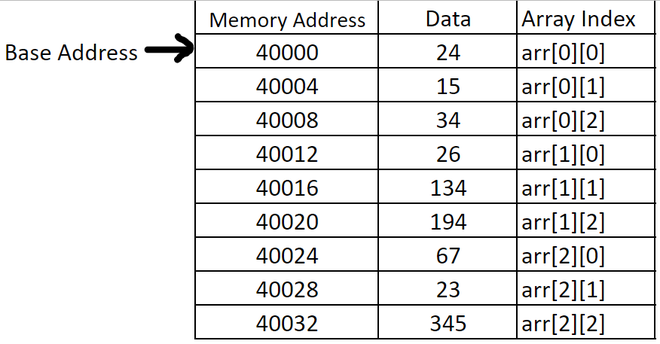Open In App

# How does C allocate memory of data items in a multidimensional array?

The data items in a multidimensional array are stored in the form of rows and columns. Also, the memory allocated for the multidimensional array is contiguous. So the elements in multidimensional arrays can be stored in linear storage using two methods i.e., row-major order or column-major order.

• Row major order: In row-major order, we store the elements according to rows i.e., first, we store the elements in the first row followed by the second row, and so on. Hence elements of the first row are stored linearly in the memory followed by the second row and so on. In memory, we will not find any separation between the rows.
• Column major order: Column major order is opposite to row-major in storing the data items i.e., Here, we first store the elements in the first column followed by the second column, and so on. So in this case, elements of the first column are stored linearly in the memory followed by the second column, and so on.

C compilers use Row major order method to store the elements in the memory. Basically, C compilers stores multidimensional arrays as single dimension arrays whose elements are single-dimensional arrays or a multidimensional array whose dimension is 1 less than the former. And the storage of these array objects is row-wise.

Size and the memory allocation of the multidimensional array:

The size of every dimension in the multidimensional array needs to be declared initially. And then the total size of the multidimensional array is calculated by multiplying the size of each dimension. And the memory allocated to the multidimensional array is equal to multiple of size of the multidimensional array and the size of a single element

data_type array_name [ x1 ] [ x2 ] [ x3 ] ….. [ xn ] ;
size of the multidimensional array :- x1*x2*x3*…..*xn
where x1, x2, x3, …, xn are the sizes of each dimensions.
memory allocated = size of the multidimensional array * size of single data_type

For example:-
int arr [ 3 ][ 4 ] [ 2 ];
size of this multidimensional (3 D) array is 3*4*2 = 24
memory allocated = 24 * 4 bytes = 96 bytes

For Example:

As given below: int arr  = { {24, 15, 34}, {26, 134, 194}, {67, 23, 345} } is stored in memory as:-Allocation of data

Here, the base address is the address of the multidimensional array or the address of the first object stored in the multidimensional array. The memory address increases by 4 bytes (the size of the int data type).

Below is the Implementation of the above concept:

## C

 `// C Program to show allocation of data items``// in multidimensional array` `#include ``#include ` `int` `main()``{` `    ``// Declaring the dimension of``    ``// 2-Dimension array``    ``int` `row = 3;``    ``int` `col = 3;` `    ``// Creating an array arr with size row*col``    ``int``* arr = (``int``*)``malloc``(row * col * ``sizeof``(``int``));``    ``int` `k = 1;` `    ``// Inserting values in the array``    ``for` `(``int` `i = 0; i < row; i++) {``        ``for` `(``int` `j = 0; j < col; j++) {` `            ``// The memory address of arr[i][j]``            ``// is equal to (arr + i*col + j)``            ``*(arr + i * col + j) = k;``            ``k++;``        ``}``    ``}` `    ``// Printing the multidimensional array``    ``for` `(``int` `i = 0; i < row; i++) {``        ``for` `(``int` `j = 0; j < col; j++) {``            ``printf``(``"%d "``, *(arr + i * col + j));``        ``}``        ``printf``(``"\n"``);``    ``}``    ``return` `0;``}`

Output

```1 2 3
4 5 6
7 8 9 ```

Time Complexity: O(R*C), where R and C are number of Rows and Columns respectively.
Auxiliary Space: O(R*C)

The above code implementation shows how elements are stored linearly in row-major order.